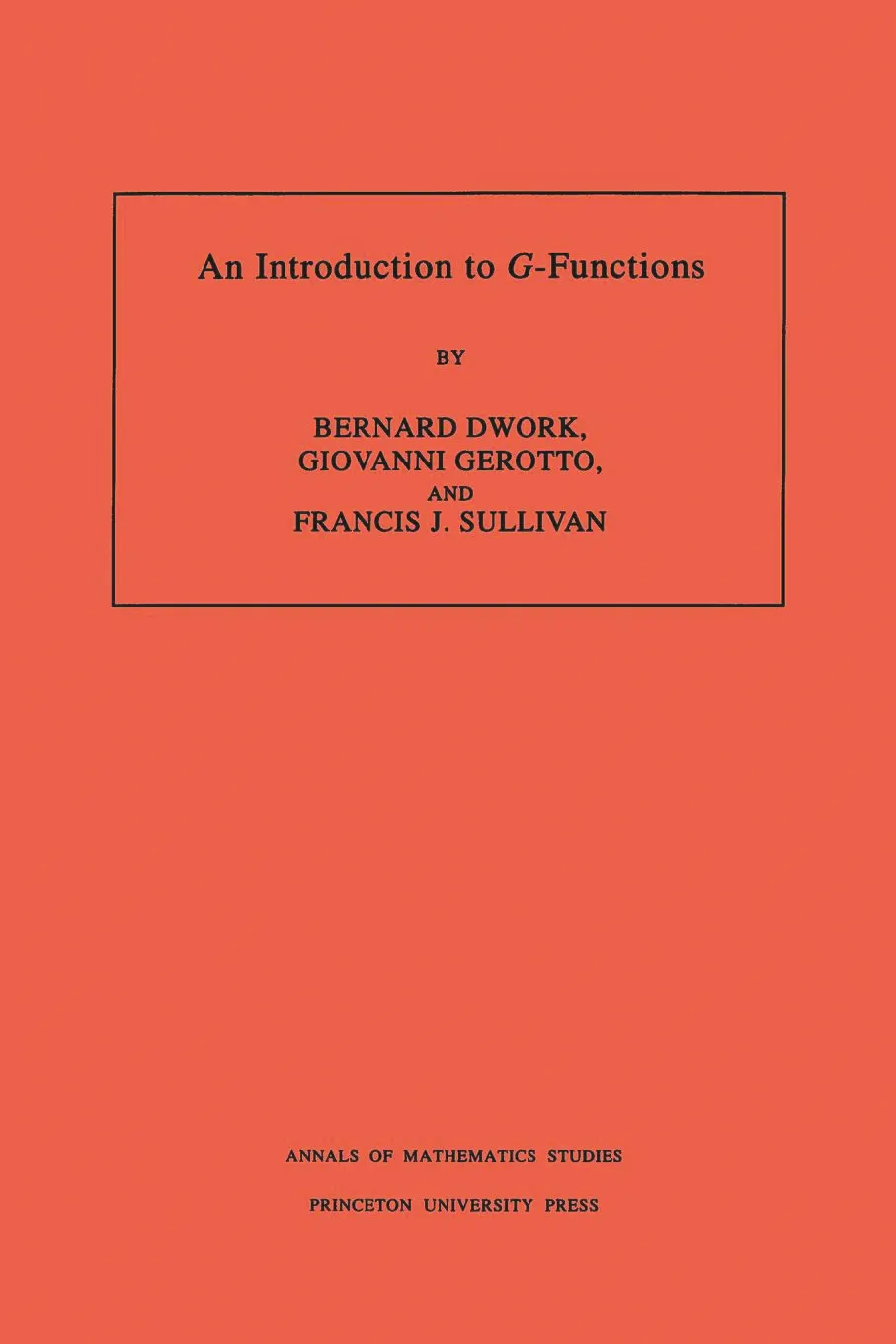#### An Introduction to G-Functions. (AM-133), Volume 133

Bernard Dwork

##### ebooks

Many of our ebooks are available for purchase from these online vendors:

Many of our ebooks are available through library electronic resources including these platforms:

# An Introduction to G-Functions. (AM-133), Volume 133## Paperback

Price:
\$105.00 / £82.00
ISBN:
Published:
May 22, 1994
1994
Pages:
352
Size:
7.75 x 10 in.
Illus:
22 figs.

Written for advanced undergraduate and first-year graduate students, this book aims to introduce students to a serious level of p-adic analysis with important implications for number theory. The main object is the study of G-series, that is, power series y=aij=0 Ajxj with coefficients in an algebraic number field K. These series satisfy a linear differential equation Ly=0 with LIK(x) [d/dx] and have non-zero radii of convergence for each imbedding of K into the complex numbers. They have the further property that the common denominators of the first s coefficients go to infinity geometrically with the index s.

After presenting a review of valuation theory and elementary p-adic analysis together with an application to the congruence zeta function, this book offers a detailed study of the p-adic properties of formal power series solutions of linear differential equations. In particular, the p-adic radii of convergence and the p-adic growth of coefficients are studied. Recent work of Christol, Bombieri, André, and Dwork is treated and augmented. The book concludes with Chudnovsky’s theorem: the analytic continuation of a G -series is again a G -series. This book will be indispensable for those wishing to study the work of Bombieri and André on global relations and for the study of the arithmetic properties of solutions of ordinary differential equations.